## Monday, 16 December 2013

### Density Plot - lattice package

One of the packages that offer more advanced graph options is 'lattice' package. One of the useful plots in this package is densityplot() function which, as the name suggest, displays density of the distribution of values of the selected variable. The parameters used within densityplot() function is identical to those of xyplot(). However, the parameter 'type' used in xyplot() is irrelevant in this function, as there is only one type (i.e. lines) to represent density. Furthermore, x-axis needs to be a numerical variable.

The below is an example of a basic density plot.

library(lattice) densityplot(~yield, barley)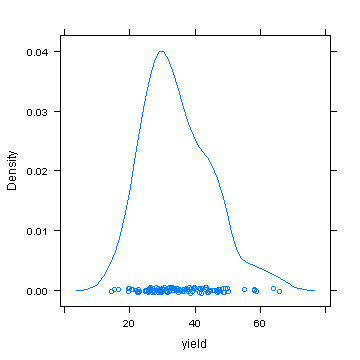If you want to hide points and only show lines in the density plot, set plot.points to 'FALSE'.

densityplot(~yield, barley, plot.points=FALSE)

Changing graphic elements, title and subtitle is done in the same way as it would be done in xyplot().

densityplot(~yield, barley, lty = 3, lwd = 2, col = "violet", main = "Amount of Barley Yield", sub = "Density Plot", par.settings = list(par.main.text = list(cex = 1.8, col = "blue"), par.sub.text = list(cex = 1.2, col = "green"), par.xlab.text = list(cex = 0.8, col = "purple"), par.ylab.text = list(cex = 0.8, col = "steelblue")))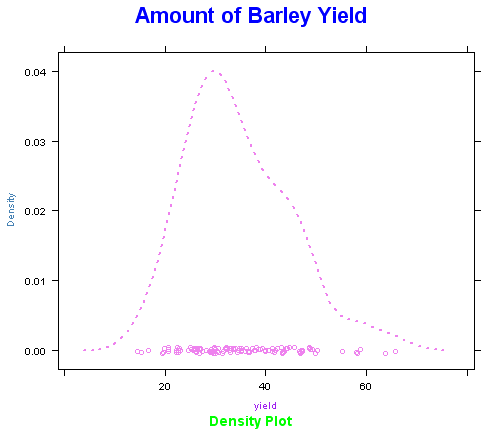The below is an example of drawing a number of density plots in multiple panes of a single graphic output. If the variable used in splitting the pane is numerical, use as.character() to convert it to categorical.

densityplot(~yield | variety, barley)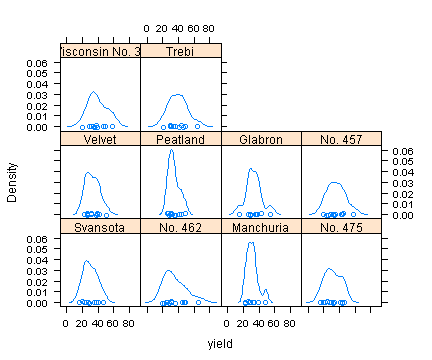To reorganise the appearance of the panes, use 'layout' parameter.

densityplot(~yield | variety, barley, layout = c(2, 5))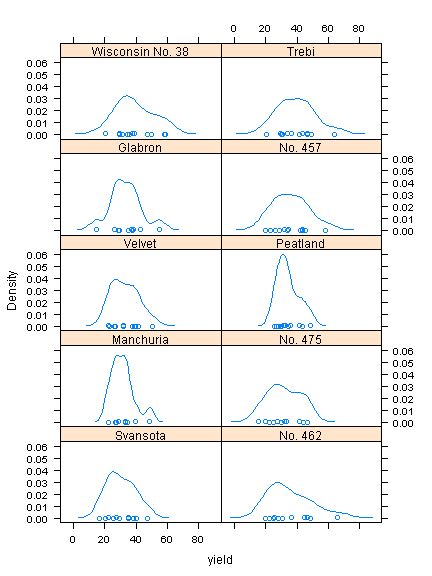When grouping values within the density plot, use 'groups' parameter.

densityplot(~yield | variety, barley, layout = c(2, 5), groups = barley\$year)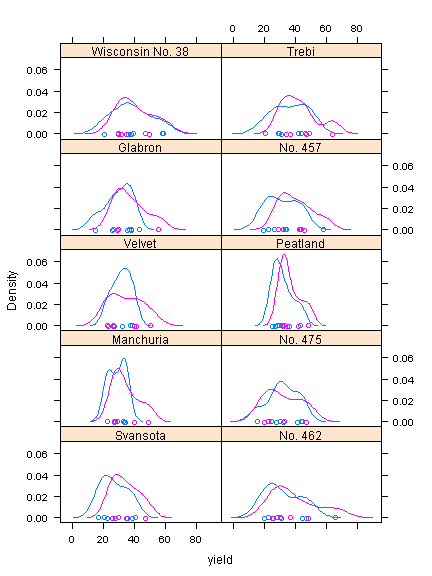The below is an example of adding legend or keys to density plot. The parameters 'size' refers to size of the symbol; 'cex' refers to size of the label, and 'between' refers to the gap between the symbol and label.

densityplot(~yield | variety, barley, layout = c(2, 5), groups = barley\$year, auto.key = list(space = "right", cex = 0.7, size = 2, lines = TRUE, between = 0.5))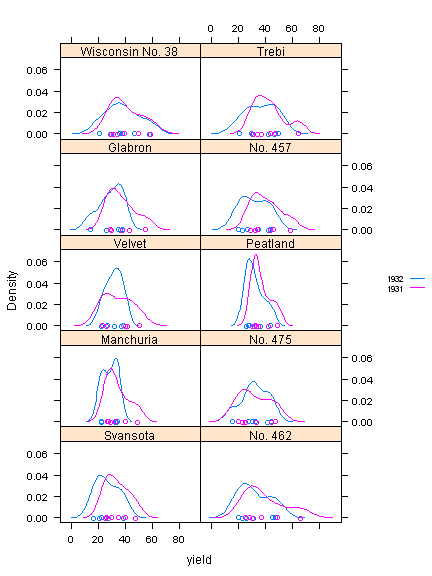When calculating density, the unit area needs to be defined. In densityplot(), which is 2 dimensional, uses unit distance along x-axis to calculate the density. In the function, the parameter that determines this unit area/distance is 'n'. The default value of 'n' is 50, and the below example shows how the above graph changes when 'n' is set to 10. It can be seen that the lines are not so smooth any more.

densityplot(~yield | variety, barley, layout = c(2, 5), groups = barley\$year, auto.key = list(space = "right", cex = 0.7, size = 2, lines = TRUE, between = 0.5), n = 10)